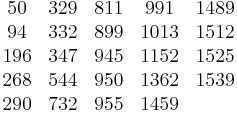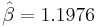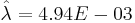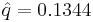# Example: Parametric RDA - Air Condition Unit

This example appears in the Life Data Analysis Reference book.

The following table gives the failure times for the air conditioning unit of an aircraft. The observation ended by the time the last failure occurred, as discussed in Cox .$\begin{matrix} \text{50} & \text{329} & \text{811} & \text{991} & \text{1489} \\ \text{94} & \text{332} & \text{899} & \text{1013} & \text{1512} \\ \text{196} & \text{347} & \text{945} & \text{1152} & \text{1525} \\ \text{268} & \text{544} & \text{950} & \text{1362} & \text{1539} \\ \text{290} & \text{732} & \text{955} & \text{1459} & {} \\ \end{matrix}\,\!$

1. Estimate the GRP model parameters using the Type I virtual age option.

2. Plot the failure number and instantaneous failure intensity vs. time with 90% two-sided confidence bounds.

3. Plot the conditional reliability vs. time with 90% two-sided confidence bounds. The mission start time is 40 and mission time is varying.

4. Using the QCP, calculate the expected failure number and expected instantaneous failure intensity by time 1800.

Solution

Enter the data into a parametric RDA folio in Weibull++. On the control panel, select the 3 parameters option and the Type I setting. Keep the default simulation settings. Click Calculate.

1. The estimated parameters are$\hat{\beta }=1.1976\,\!$,$\hat{\lambda }=4.94E-03\,\!$,$\hat{q}=0.1344\,\!$.
2. The following plots show the cumulative number of failures and instantaneous failure intensity, respectively.

3. The following plot shows the conditional reliability.
4. Using the QCP, the failure number and instantaneous failure intensity are: